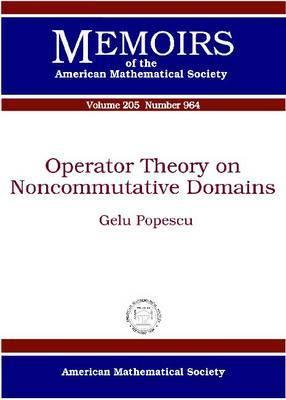Home » Operator Theory on Noncommutative Domains by Gelu Popescu# Operator Theory on Noncommutative Domains

## Gelu Popescu

Published September 21st 2010
ISBN : 9780821847107
Paperback
124 pages
Book Rating:Enter the sum

 About the Book In this volume the author studies noncommutative domains \$/mathcal{D}_f/subset B(/mathcal{H}) DEGREESn\$ generated by positive regular free holomorphic functions \$f\$ on \$B(/mathcal{H}) DEGREESn\$, where \$B(/mathcal{H})\$ is the algebra of all boundedMoreIn this volume the author studies noncommutative domains \$/mathcal{D}_f/subset B(/mathcal{H}) DEGREESn\$ generated by positive regular free holomorphic functions \$f\$ on \$B(/mathcal{H}) DEGREESn\$, where \$B(/mathcal{H})\$ is the algebra of all bounded linear operators on a Hilbert space \$/mathcal{H}\$. Table of Contents: Introduction- Operator algebras associated with noncommutative domains- Free holomorphic functions on noncommutative domains- Model theory and unitary invariants on noncommutative domains- Commutant lifting and applications- Bibliography.